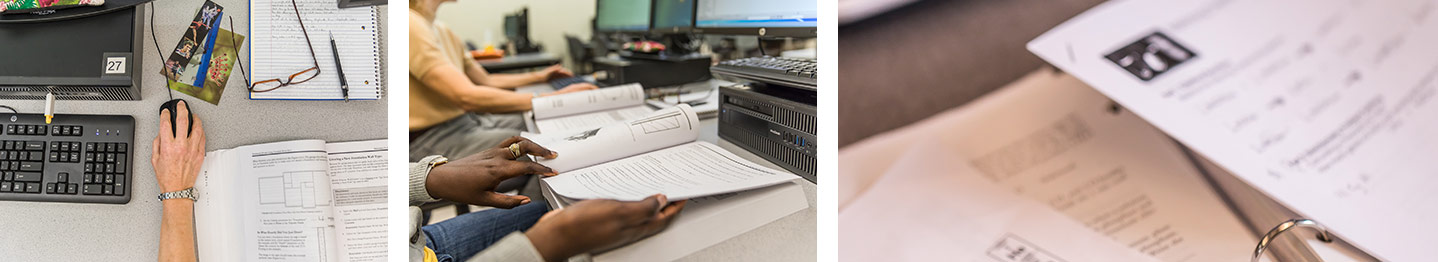Mathematics
Program Learning Outcomes

Program Learning Outcomes detail the knowledge, skills and abilities that you as a student will demonstrate upon successful completion of your program.

#### Mathematics, College Level

1. define arithmetic, algebraic, geometric, spatial, and statistical concepts

2. calculate arithmetic, algebraic, geometric, spatial, and statistical quantities using appropriate technology.

3. estimate arithmetic, algebraic, geometric, spatial, and statistical solutions

4. solve arithmetic, algebraic, geometric, spatial, and statistical expressions, equations, functions, and problems using appropriate technology.

5. represent mathematical information numerically, symbolically, graphically, verbally, and visually using appropriate technology.

6. develop mathematical and statistical models such as formulas, functions, graphs, tables, and schematics using appropriate technology.

7. Interpret mathematical and statistical models such as formulas, functions, graphs, tables, and schematics, drawing conclusions and making inferences based on those models.

8. Explore mathematical systems utilizing rich experiences that encourage independent, nontrivial, constructive exploration in mathematics.

9. Communicate mathematical thoughts and ideas clearly and concisely to others in the oral and written form.

Associated Degrees

Department Coordinator
Edgar Jasso
email
(206) 934-4516

Math & Science Division

Location
IB 2429

Division Contacts
(206) 934-3746
(206) 934-3748 (fax)